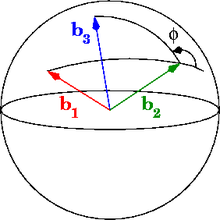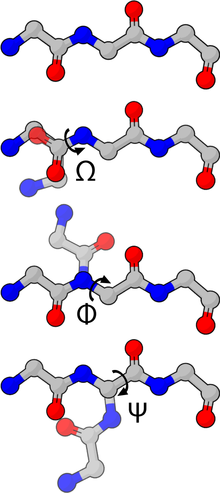# Dihedral angle

﻿
Dihedral angle
In aerospace engineering, the dihedral is the angle between the two wings; see Dihedral (aircraft).

In geometry, a dihedral or torsion angle is the angle between two planes.Figure 1: Dihedral angle of three vectors, defined as an exterior spherical angle. The longer and shorter black segments are arcs of the great circles passing through$\mathbf{b}_{1}$ and$\mathbf{b}_{2}$ and through$\mathbf{b}_{2}$ and$\mathbf{b}_{3}$, respectively.Figure 2: Dihedral angle defined by three bond vectors (shown in red, green and blue) connecting four atoms.Figure 3: Dihedral angle defined by three bond vectors (shown in red, green and blue) connecting four atoms. From this perspective, the second bond vector (green) is coming out of the page.Figure 4: The backbone dihedral angles of proteins.

The dihedral angle of two planes can be seen by looking at the planes "edge on", i.e., along their line of intersection. The dihedral angle φAB between two planes denoted A and B is the angle between their two normal unit vectors$\mathbf{n}_{A}$ and$\mathbf{n}_{B}:$$\cos \varphi_{AB} = \mathbf{n}_A \cdot \mathbf{n}_B.$

A dihedral angle can be signed; for example, the dihedral angle φAB can be defined as the angle through which plane A must be rotated (about their common line of intersection) to align it with plane B. Thus, φAB = − φBA. For precision, one should specify the angle or its supplement, since both rotations will cause the planes to coincide.

## Alternative definitions

Since a plane can be defined in several ways (e.g., by vectors or points in them, or by their normal vectors), there are several equivalent definitions of a dihedral angle.

Any plane can be defined by two non-collinear vectors lying in that plane; taking their cross product and normalizing yields the normal unit vector to the plane. Thus, a dihedral angle can be defined by four, pairwise non-collinear vectors.

We may also define the dihedral angle of three non-collinear vectors$\mathbf{b}_{1}$,$\mathbf{b}_{2}$ and$\mathbf{b}_{3}$ (shown in red, green and blue, respectively, in Figure 1). The vectors$\mathbf{b}_{1}$ and$\mathbf{b}_{2}$ define the first plane, whereas$\mathbf{b}_{2}$ and$\mathbf{b}_{3}$ define the second plane. The dihedral angle corresponds to an exterior spherical angle (Figure 1), which is a well-defined, signed quantity.$\varphi = \operatorname{atan2} \left( |\mathbf{b}_2| \mathbf{b}_1 \cdot [\mathbf{b}_2 \times \mathbf{b}_3], [\mathbf{b}_1 \times \mathbf{b}_2] \cdot [\mathbf{b}_2 \times \mathbf{b}_3] \right)$

where the two-argument atan2 takes care of the sign.

## Dihedral angles in polyhedra

Every polyhedron, regular and irregular, convex and concave, has a dihedral angle at every edge.

A dihedral angle (also called the face angle) is the internal angle at which two adjacent faces meet. An angle of zero degrees means the face normal vectors are antiparallel and the faces overlap each other (Implying part of a degenerate polyhedron). An angle of 180 degrees means the faces are parallel (like a tiling). An angle greater than 180 exists on concave portions of a polyhedron.

Every dihedral angle in an edge-transitive polyhedron has the same value. This includes the 5 Platonic solids, the 4 Kepler–Poinsot polyhedra, the two quasiregular solids, and two quasiregular dual solids.

## Dihedral angles of four atoms

The structure of a molecule can be defined with high precision by the dihedral angles between three successive chemical bond vectors (Figure 2). The dihedral angle φ varies only the distance between the first and fourth atoms; the other interatomic distances are constrained by the chemical bond lengths and bond angles.

To visualize the dihedral angle of four atoms, it's helpful to look down the second bond vector (Figure 3), which is equivalent to the Newman projection in chemistry. The first atom is at 6 o'clock, the fourth atom is at roughly 2 o'clock and the second and third atoms are located in the center. The second bond vector is coming out of the page. The dihedral angle φ is the counterclockwise angle made by the vectors$\mathbf{b}_{1}$ (red) and$\mathbf{b}_{3}$ (blue). When the fourth atom eclipses the first atom, the dihedral angle is zero; when the atoms are exactly opposite (as in Figure 2), the dihedral angle is 180°.

## Dihedral angles of biological molecules

The backbone dihedral angles of proteins are called φ (phi, involving the backbone atoms C'-N-Cα-C'), ψ (psi, involving the backbone atoms N-Cα-C'-N) and ω (omega, involving the backbone atoms Cα-C'-N-Cα). Thus, φ controls the C'-C' distance, ψ controls the N-N distance and ω controls the Cα-Cα distance.

The planarity of the peptide bond usually restricts ω to be 180° (the typical trans case) or 0° (the rare cis case). The distance between the Cα atoms in the trans and cis isomers is approximately 3.8 and 2.9 Å, respectively. The cis isomer is mainly observed in Xaa-Pro peptide bonds (where Xaa is any amino acid).

The sidechain dihedral angles of proteins are denoted as χ15, depending on the distance up the sidechain. The χ1 dihedral angle is defined by atoms N-Cα-Cβ-Cγ, the χ2 dihedral angle is defined by atoms Cα-Cβ-Cγ-Cδ, and so on.

The sidechain dihedral angles tend to cluster near 180°, 60°, and −60°, which are called the trans, gauche+, and gauche- conformations. The choice of sidechain dihedral angles is affected by the neighbouring backbone and sidechain dihedrals; for example, the gauche+ conformation is rarely followed by the gauche+ conformation (and vice versa) because of the increased likelihood of atomic collisions.

Dihedral angles have also been defined by the IUPAC for other molecules, such as the nucleic acids (DNA and RNA) and for polysaccharides.

## Methods of computation

The dihedral angle between two planes relies on being able to efficiently generate a normal vector to each of the planes. One approach is to use the cross product. If A1, A2, and A3 are three non-collinear points on plane A, and B1, B2, and B3 are three non-collinear points on plane B, then UA = (A2A1) × (A3A1) is orthogonal to plane A and UB = (B2B1) × (B3B1) is orthogonal to plane B. The (unsigned) dihedral angle can therefore be computed with either$\varphi_{AB}=\arccos \left(\frac{|U_A \centerdot U_B|}{|U_A| |U_B|}\right) = \arcsin \left(\frac{|U_A \times U_B|}{|U_A| |U_B|}\right).$

Another approach to computing the dihedral angle is first to pick an arbitrary vector V that is not tangent to either of the two planes. Then applying the Gram–Schmidt process to the three vectors (A2A1, A3A1, V) produces an orthonormal basis of space, the third vector of which will be normal to plane A. Doing the same with the vectors (B2B1, B3B1, V) yields a vector normal to plane B. The angle between the two normal vectors can then be computed by any method desired. This approach generalizes to higher dimensions, but does not work with flats that have a codimension greater than 1.

To compute the dihedral angle between two flats, it is additionally necessary to ensure that each of the two normal vectors is selected to have a minimal projection onto the other flat. The Gram–Schmidt process does not guarantee this property, but it can be guaranteed with a simple eigenvector technique. If$\mathbf{A}$ is a matrix of orthonormal basis vectors for flat A, and$\mathbf{B}$ is a matrix of orthonormal basis vectors for flat B, and
u = the eigenvector with the smallest corresponding eigenvalue of$(\mathbf{B}^T\mathbf{A})^T(\mathbf{B}^T\mathbf{A})$, and
v = the eigenvector with the smallest corresponding eigenvalue of$(\mathbf{A}^T\mathbf{B})^T(\mathbf{A}^T\mathbf{B})$,

then, the angle between u and v is the dihedral angle between A and B, even if A and B have a codimension greater than 1.

Wikimedia Foundation. 2010.

### Look at other dictionaries:

• Dihedral angle — Dihedral Di*he dral, a. [Gr. di = di s twice + ? a seat, bottom, base, fr. ? to sit. Cf. {Diedral}.] 1. Having two plane faces; as, the dihedral summit of a crystal. [1913 Webster] 2. Of a kite or an a[ e]roplane, having wings that make with one… …   The Collaborative International Dictionary of English

• Dihedral Angle — Angle diédral En géométrie, l angle entre 2 plans est appelé angle diédral ou angle dièdre. L angle diédral d un tétraèdre régulier, correspond à l angle observé au sol, dans un plan perpendiculaire à l une des arêtes au sol, que font la base du… …   Wikipédia en Français

• dihedral angle — noun 1. : the angle between two intersecting planes 2. : dihedral II 2 * * * Geom. 1. the angle between two planes in a dihedron. 2. dihedron. [1820 30] * * * dihedral angle noun The angle made by the wing of an aeroplane wi …   Useful english dictionary

• dihedral angle — dvisienis kampas statusas T sritis chemija apibrėžtis Kampas tarp plokštumų, kertančių du bendrus atomus ir du ryšius prie skirtingų atomų. atitikmenys: angl. dihedral angle; torsion angle; torsional angle rus. двугранный угол; торсионный угол …   Chemijos terminų aiškinamasis žodynas

• dihedral angle — Geom. 1. the angle between two planes in a dihedron. 2. dihedron. [1820 30] * * * …   Universalium

• dihedral angle — noun The angle between two planes …   Wiktionary

• dihedral angle — dihe′dral an′gle n. math. the angle between two planes in a dihedron …   From formal English to slang

• dihedral angle — noun Etymology: di + hedral Date: 1826 a figure formed by two intersecting planes …   New Collegiate Dictionary

• Dihedral — or polyhedral may refer to: Dihedral angle, the angle between two mathematical planes Dihedral (aircraft), the upward angle of a fixed wing aircraft s wings where they meet at the fuselage, dihedral effect of an aircraft, longitudinal dihedral… …   Wikipedia

• Dihedral (disambiguation) — Dihedral may refer to: *Dihedral the angle of a fixed wing aircraft s wings *Dihedral angle, is the angle between two planes, in geometry *Dihedral group, is the group of symmetries of the n sided polygon in abstract algebra **Also Dihedral… …   Wikipedia